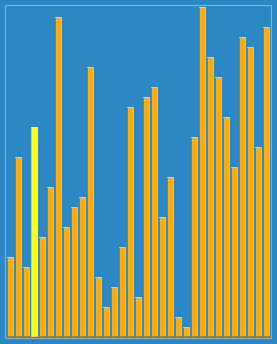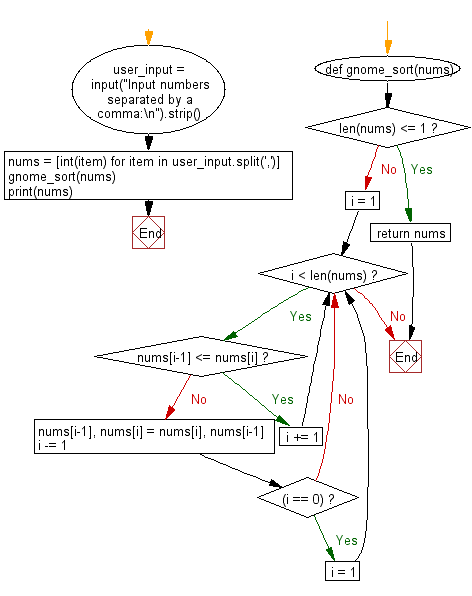﻿ Python: Sort a list of elements using Gnome sort - w3resource# Python: Sort a list of elements using Gnome sort

## Python Search and Sorting : Exercise-13 with Solution

Write a Python program to sort a list of elements using Gnome sort.
Gnome sort is a sorting algorithm originally proposed by Dr. Hamid Sarbazi-Azad (Professor of Computer Engineering at Sharif University of Technology) in 2000 and called "stupid sort" (not to be confused with bogosort), and then later on described by Dick Grune and named "gnome sort". The algorithm always finds the first place where two adjacent elements are in the wrong order, and swaps them. It takes advantage of the fact that performing a swap can introduce a new out-of-order adjacent pair only next to the two swapped elements.

A visualization of the gnome sort:Sample Solution:

Python Code:

``````def  gnome_sort(nums):
if len(nums) <= 1:
return nums

i = 1

while i < len(nums):
if nums[i-1] <= nums[i]:
i += 1
else:
nums[i-1], nums[i] = nums[i], nums[i-1]
i -= 1
if (i == 0):
i = 1

user_input = input("Input numbers separated by a comma:\n").strip()
nums = [int(item) for item in user_input.split(',')]
gnome_sort(nums)
print(nums)
```
```

Sample Output:

```Input numbers separated by a comma:
5, 79, 35, 68, 25
[5, 25, 35, 68, 79]
```

Flowchart:Python Code Editor:

What is the difficulty level of this exercise?

Test your Programming skills with w3resource's quiz.

﻿

## Python: Tips of the Day

Use Reversed() In for Loops:

```>>> tasks = ['laundry', 'picking up kids', 'gardening', 'cooking']